Image credit: Gimages

# GAN 5

Conditional GAN

As we have seen in GAN 1, GAN 2, GAN 3, GAN 4 that GANs have 2 network, the Generator G and the Discriminator D. Given a latent vector z, the G generates a new sample from the distribution of the training data. D classifies a data sample as real(from the training data) or fake(generated by G).In the starting, the G generates a random data sample(as it didnt learn the data distribution) and the D is not a good classifier now. As the training process goes, the G starts learning the data distribution and D becomes a good classifier. D tries to classify all sampels generated by D as fake, G tries to generate samples such that D classifies that as real. In the process, both the networks become better and Generator learns the distribution of the data and can now generate realistic samples. D becomes good at classifying real/fake data samples.# Conditional GAN

Let us consider MNIST GAN, after we trained a MNIST dataset on a GAN model, the generator(G) can now generate some images which look alike of the MNIST numbers.But what if we want the G to generate images of a specific digit?. The G which we trained generated images samples depending on the latent vector z. But we used a random z. So we cannot choose a map from random z - > Specific image.

So we introduce a conditional label y, such that for a condition label y the generator have to generate sample.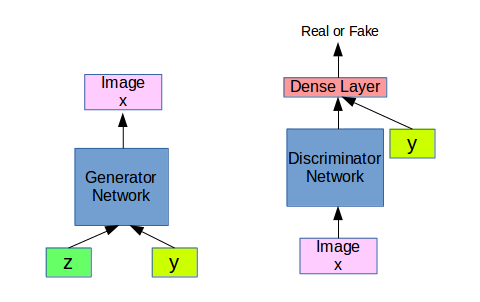Now the Generator learns the distribution of the dataset and generates samples based on the condition y or c(condition).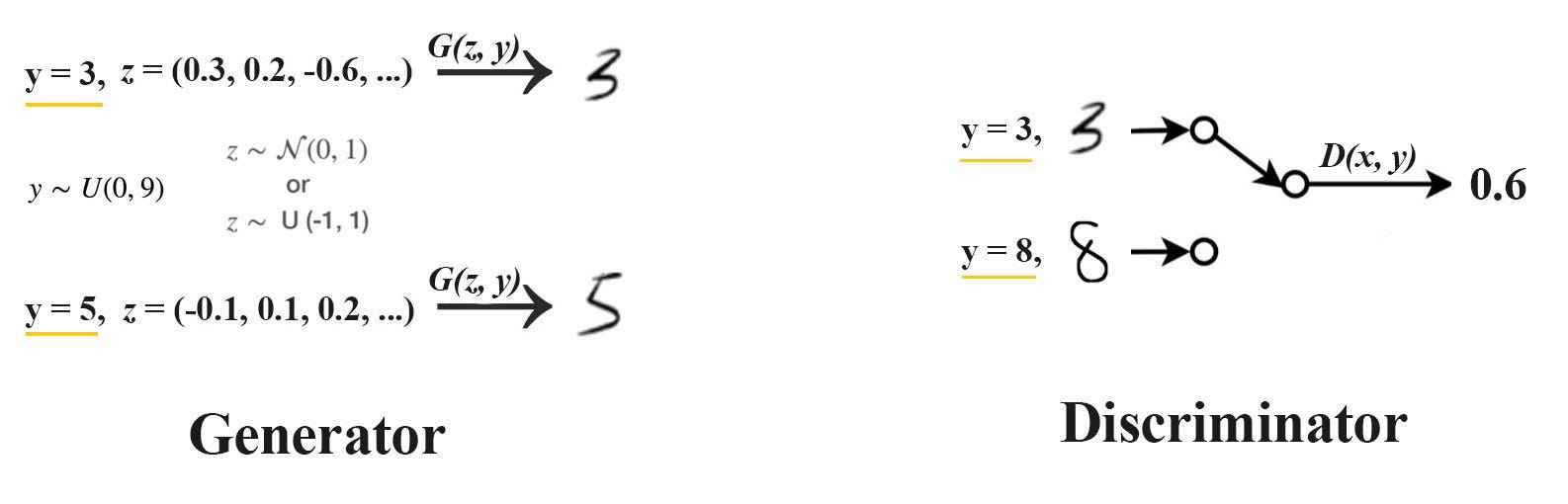The representation may vary, but the concept is the same.

We can also generate a output for a specific input, G : x -> y. Here we generate an image y given an inpu image x. This is a Pix2Pix GAN.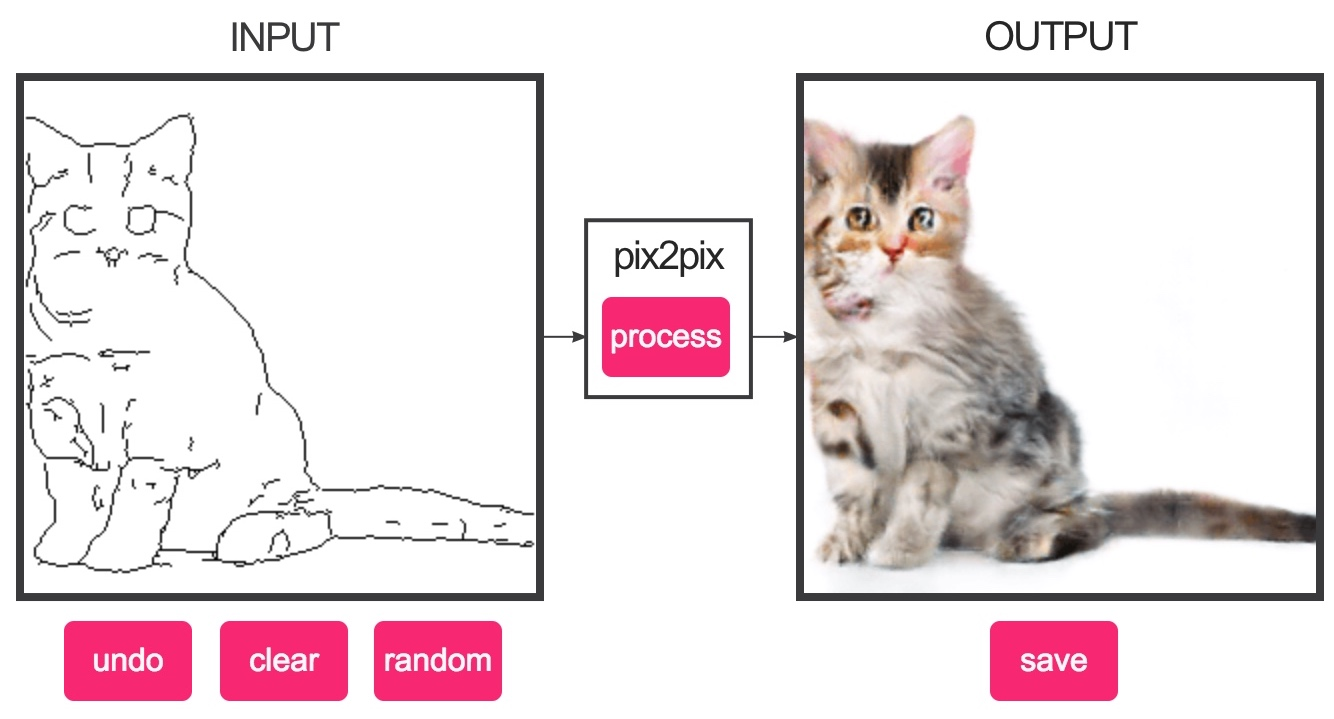Check this amazing demo of Pix2Pix GAN

This kind of image to image (pix2pix) can be done with the help of Encoder-Decoder architecture, where the input image is encoded to a feature representation vector anf this vector is decoded to the target image.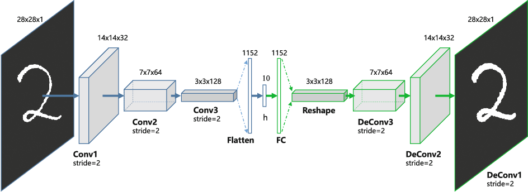So the generator will learn the mapping from G: x->y with autoencoder architecture, and generate new samples for the given x.

The generator G will get a pair of images:

• training x and training y\
G will classify as real
• training x and generated y(for x)\
G will classify as fake
• training x and generated y (different x)\
G will classify as fake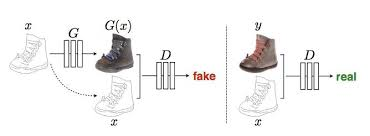This way a conditional GAN(CGAN) or pix2pix GAN is trained, which has massive applications. In the next post we will see how to train a GAN to do a image to image translation(pix2pix) without labelled pair.##### Shangeth Rajaa
###### Researcher at Vernacular.ai

Machine Learning Researcher at Vernacular.ai